JAIC 1993, Volume 32, Number 1, Article 7 (pp. 81 to 92)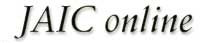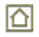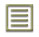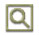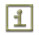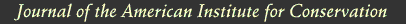JAIC 1993, Volume 32, Number 1, Article 7 (pp. 81 to 92)

### 3.1 DESIGN OF APPARATUS AND CALCULATION OF DIFFUSION CONSTANT

Diffusion measurements were made following the general design used in obtaining diffusion constants for gases in porous media (Smith 1981). In these experiments a feed gas containing a fixed concentration of pollutants in air was passed on one side of the boxboard. On the other side of the sample, air (no pollutants) at the same RH was passed as a sweep gas. The concentration of pollutant in each chamber was measured, and these concentrations were used with the flow rate and sample geometry to calculate the diffusion constant. Figure 1 shows the design of the diffusion cell.

The following section indicates how the diffusion constant may be determined. The flow of the pollutant across the paper (Smith 1981; Barrer 1941; Crank and Park 1968) is: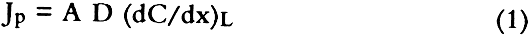where Jp is the flow of the pollutant across the boxboard, A is the cross-sectional area of the boxboard exposed to the gas, D is the diffusion constant, and (dC/dx)L is the change in concentration of SO2 as a function of distance at the edge of the boxboard in the chamber into which the air is introduced. The pollutant flow may also be expressed by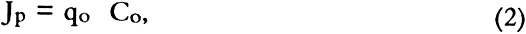where Co is the concentration of SO2 in the outlet side of the chamber where air is introduced and qo is the flow rate of gas in that chamber. If we assume that, at steady state, the concentration across the boxboard is linear (Crank 1975) then,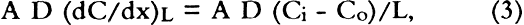where Ci is the concentration of SO2 on the top compartment of the apparatus (see fig. 1) and L is the thickness of the boxboard. Therefore,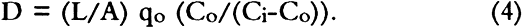This treatment neglects the possibility of SO2 dissolution by the boxboard, and if this occurs in our samples, we may be measuring permeability constants rather than diffusion constants. No suitable treatment was found in the literature to describe diffusion of a pollutant through a porous medium where the pollutant dissolves in the medium. However, since the change of concentration of SO2 in the stacks surrounding archival boxes is expected to be slow (Baer and Banks 1985; Passaglia 1987), our calculated diffusion constant should be a good measure of mass flow of pollutants into archival boxes. For the rest of this article we shall assume the calculated quantity is a diffusion constant.

Sulfur dioxide diffused through boxboards G, H, and J so slowly that a diffusion constant could not be determined in our apparatus. However, the smallest concentration that could be determined may be estimated by using the GC-FPD calibration curve for SO2. For SO2 at 147 ppm in air, this concentration gives a value of 0.0025 for Co/(Ci-Co) in equation 3. For a value of qo of 10 ml/min using a 60-point boxboard, the smallest diffusion constant that can be determined by our measurement procedure is 2 � 10-5 cm2/sec. Therefore, the diffusion constants of boxboards G, H, and J must be less than that value.

Since SO2 is absorbed by paper (Hudson et al. 1964; Edwards et al. 1968; Atherton et al. 1973), one might be concerned that no SO2 was observed diffusing through boxboards G, H, and J due to the massive absorption of SO2. Concentration of the gas before it impinged on the boxboard (CB) was routinely measured. This measurement was made to check the calibration on the GC-FPD and the mass balance in the system. During the measurement, the concentration of pollutant leaving the top chamber was not significantly different from CB for all the boxboards measured. If significant absorption of SO2 had occurred in boxboards G, H, and J, a large concentration drop would have been expected. Such a concentration drop was not found.

### 3.2 EFFECT OF BOUNDARY CONDITION ON THE VALUE OF THE DIFFUSION CONSTANT

Diffusion constants are obtained from experiments by assuming the measurements have been made on a sheet of infinite dimensions. Experimentally, that condition cannot be obtained. When diffusion through a finite area is measured, diffusion through the edges must be considered.

In a boundary condition used in many diffusion experiments, the edges of the apparatus are sealed, thereby eliminating flow in that direction (Crank 1975; Barrer et al. 1962). Barrer et al. (1962) discussed the error made by this boundary condition. For this current work, several measurements under this condition were performed. Under our experimental conditions, Barrer's modeling suggests that using this boundary condition leads to a diffusion constant that is no more than 8% larger than the diffusion constant obtained for the infinite sheet case.

Measuring the diffusion constants in an apparatus that allows the sides of the boxboard to remain open is another boundary condition. Under this condition, mass may be lost through the edge, thereby reducing the concentration in the bottom compartment. For this case, the measured diffusion constant is lower than that for the infinite sheet case.

The true (infinite sheet) diffusion constant lies between those found under these two boundary conditions. In table 2, diffusion constants obtained for various boxboards at 147 ppm SO2 in air are listed for these boundary conditions. These data indicate that the effect of boundary conditions on our measurement is generally less than 20%.

TABLE 2 EFFECT OF APPARATUS BOUNDARY CONDITION ON MEASURED DIFFUSION CONSTANT

### 3.3 ACHIEVEMENT OF STEADY STATE CONDITION

All diffusion constants were obtained after steady state had been reached, so that the effects of SO2 absorption in the boards could be minimized (Crank and Park 1968). Normally, the experiment was started approximately 24 hours before data collection was initiated. Points were then taken regularly two or three times daily. Steady state was assumed when the value of the diffusion constant showed no drift with time.

Errors in the calculated average value of the diffusion constant were estimated from the standard deviations of repeated data taken for a number of hours after steady state had been achieved. These are reported in tables 2–4. On boxboard A, measurements were made on three samples cut from different parts of the NARA box. In this case, errors estimated from the sample to sample variation in D were comparable to the errors estimated from the measurements on one board.

TABLE 3 EFFECTS OF GAS CONCENTRATION DIFFUSION CONSTANT (a)

TABLE 4 DIFFUSION CONSTANT OF VARIOUS BOXBOARDS AT 147 PPM SO2 IN AIR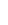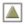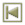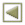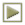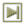Copyright � 1993 American Institute for Conservation of Historic and Artistic Works